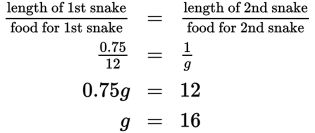# SAT Math Multiple Choice Question 416: Answer and Explanation

### Test Information

Question: 416

11. Zoos use various methods for determining how to feed different animals. Sometimes they use age, weight, or, usually in the case of snakes, length. If a snake that is 2 feet, 6 inches long receives 12 grams of frog mash per feeding, how many grams should a snake that is 1 meter in length get? (Use the approximate conversion 1 foot = 0.3 meters.)

• A. 5
• B. 13
• C. 14.5
• D. 16

Explanation:

D

Difficulty: Hard

Category: Problem Solving and Data Analysis / Rates, Ratios, Proportions, and Percentages

Strategic Advice: A question like this requires a simple proportion. However, you need to convert the units so they are the same. You can convert feet to meters or meters to feet—it doesn't matter which you choose, as long as the lengths end up in the same unit of measure.

Getting to the Answer: Because the conversion given at the end of the question is feet to meters, convert the first snake length to meters by multiplying it by 0.3. Remember, 2 feet, 6 inches is the same as 2.5 feet: 2.5 feet × 0.3 meters per foot = 0.75 meters. Now, set up a proportion and solve. Let g be the number of grams of feed needed for a snake that is 1 meter long. Write the proportion in words first to keep the pieces organized:A snake that is 1 meter long should receive 16 grams of frog mash.